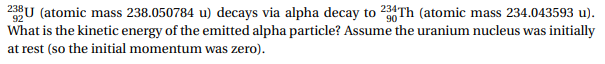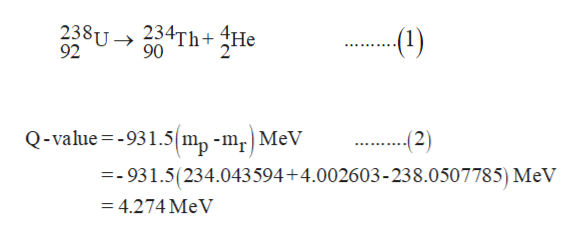# 23U (atoic mass 238.050784 u) decays via alpha decay to 234Th (atomic mass 234.043593 u).What is the kinetic energy of the emitted alpha particle? Assume the uranium nucleus was initiallyat rest (so the initial momentum was zero).

Question
14 viewshelp_outlineImage Transcriptionclose23U (atoic mass 238.050784 u) decays via alpha decay to 234Th (atomic mass 234.043593 u). What is the kinetic energy of the emitted alpha particle? Assume the uranium nucleus was initially at rest (so the initial momentum was zero). fullscreen
check_circle

Step 1

Alpha-decay of  238uranium is shown by equation (1)

Mass of uranium atom =238.0507785 u

Mass of thorium atom =234.043594 u

Mass of alpha-particles = 4.002603 u

Q-value for this reactionhelp_outlineImage Transcriptionclose234Th+4He 90 238U 92 (1) (2) Q-value-931.5 m,-mr MeV =-931.5(234.043594+4.002603-238.0507785) MeV = 4.274 MeV fullscreen
Step 2

It is given that the initial momentum of uranium is zero.

According to the conservation of momentum law-

As momentum  (P) = mass × velocity

Als...help_outlineImage TranscriptionclosePU PThPHe - Рrh-Pне 0 P ThHe =P Th (3) MThVть МНе'Не V. =__Th 'Th 4) "Не М, Не Kinetic energy (k.E)=mv2 K.E (He)2mHe"He) (5) 2 Th'Th mHe K.E(He m 2" Не Th 2 Th Th (Не Th -Κ.Ε (Th (Не K.Е(Не) 234.043594 (6) 4.002603 Κ.Ε (Th) fullscreen

### Want to see the full answer?

See Solution

#### Want to see this answer and more?

Solutions are written by subject experts who are available 24/7. Questions are typically answered within 1 hour.*

See Solution
*Response times may vary by subject and question.
Tagged in

### Atomic structure